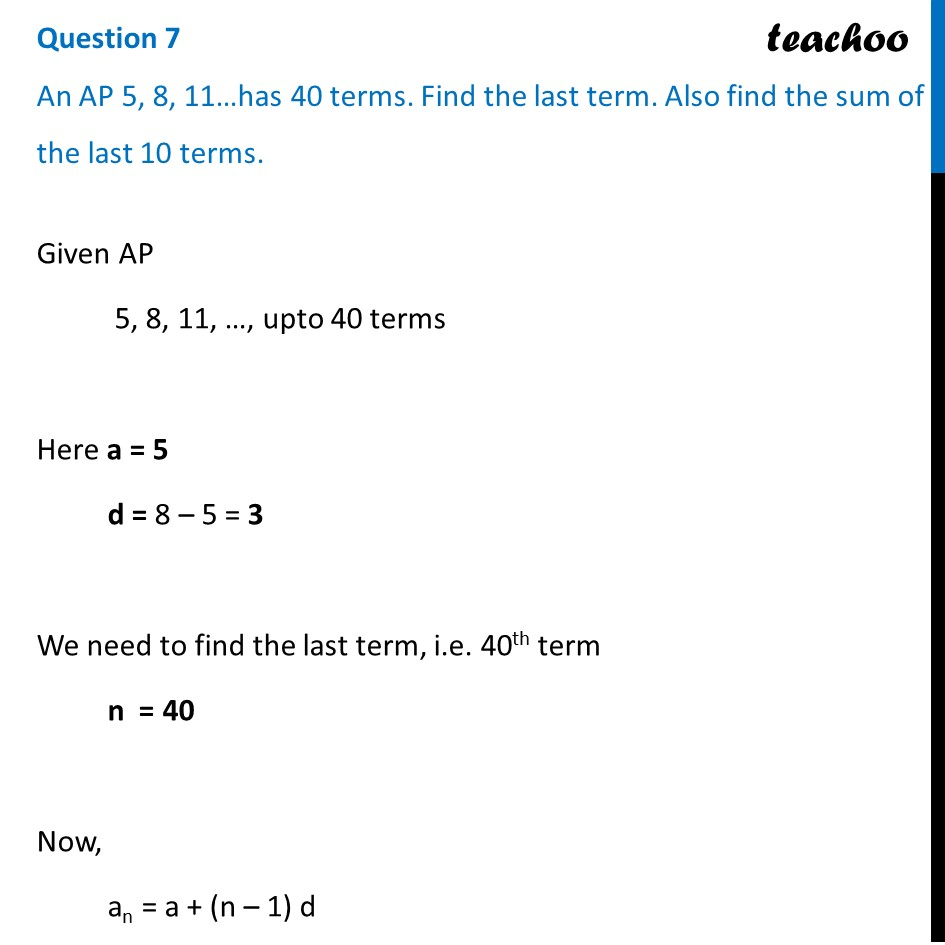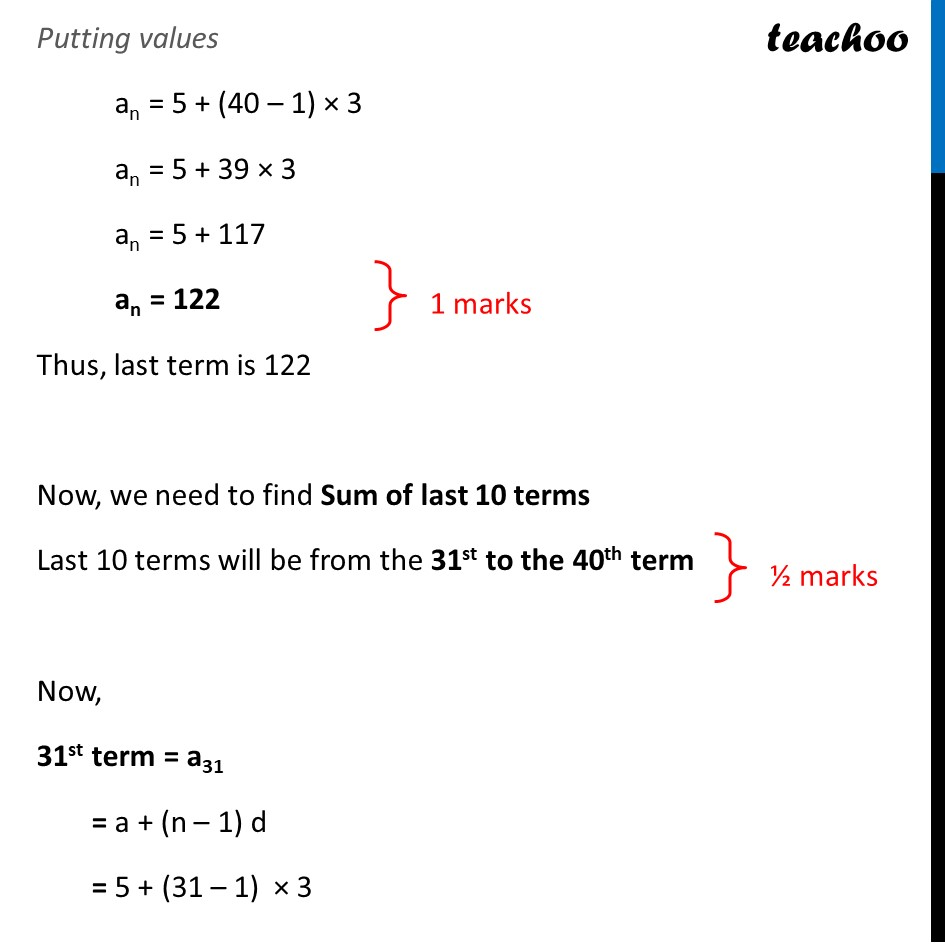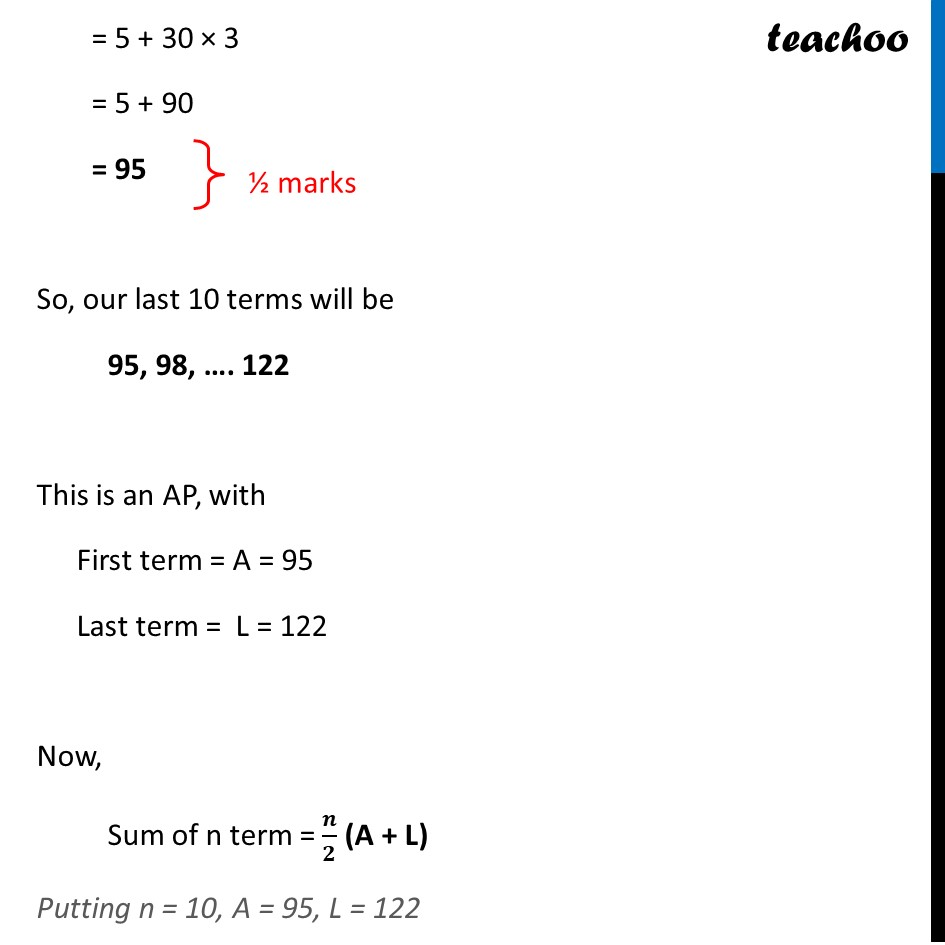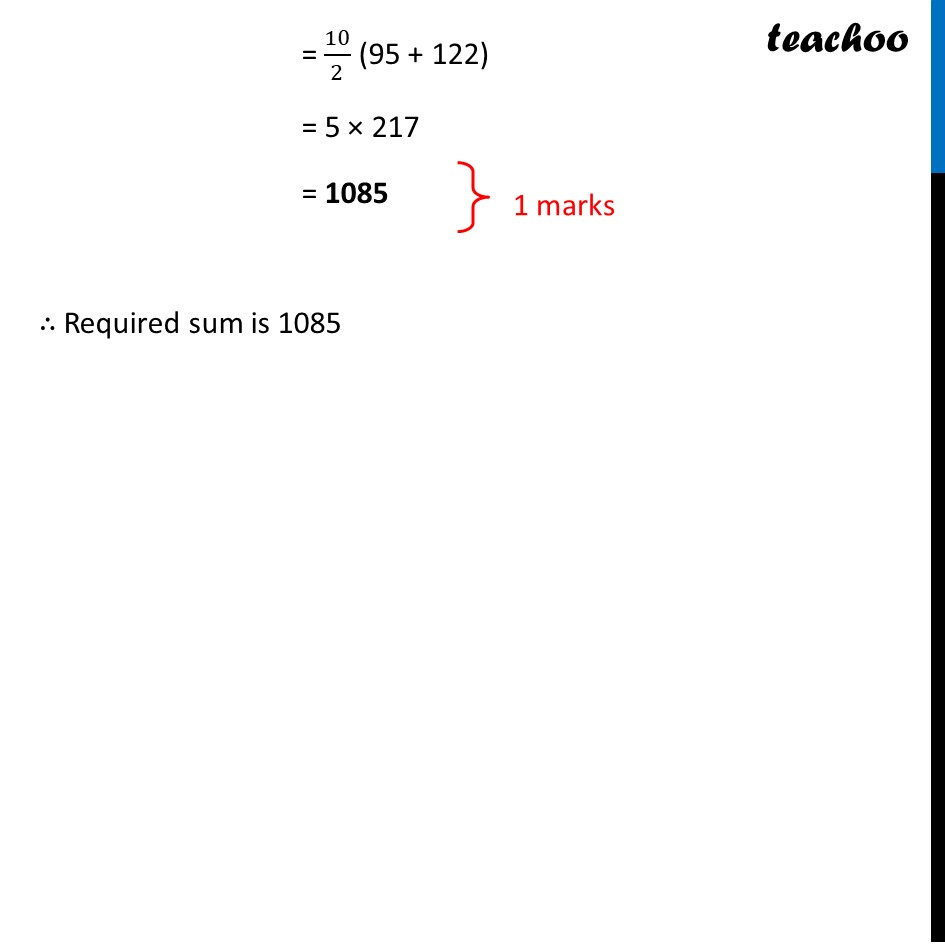CBSE Class 10 Sample Paper for 2022 Boards - Maths Basic [Term 2]

Class 10
Solutions of Sample Papers for Class 10 Boards

## An AP 5, 8, 11…has 40 terms. Find the last term. Also find the sum of the last 10 terms.Learn in your speed, with individual attention - Teachoo Maths 1-on-1 Class

### Transcript

An AP 5, 8, 11…has 40 terms. Find the last term. Also find the sum of the last 10 terms. Given AP 5, 8, 11, …, upto 40 terms Here a = 5 d = 8 – 5 = 3 We need to find the last term, i.e. 40th term n = 40 Now, an = a + (n – 1) d Putting values an = 5 + (40 – 1) × 3 an = 5 + 39 × 3 an = 5 + 117 an = 122 Thus, last term is 122 Now, we need to find Sum of last 10 terms Last 10 terms will be from the 31st to the 40th term Now, 31st term = a31 = a + (n – 1) d = 5 + (31 – 1) × 3 = 5 + 30 × 3 = 5 + 90 = 95 So, our last 10 terms will be 95, 98, …. 122 This is an AP, with First term = A = 95 Last term = L = 122 Now, Sum of n term = 𝑛/2 (A + L) Putting n = 10, A = 95, L = 122 = 10/ Putting values an = 5 + (40 – 1) × 3 an = 5 + 39 × 3 an = 5 + 117 an = 122 Thus, last term is 122 Now, we need to find Sum of last 10 terms Last 10 terms will be from the 31st to the 40th term Now, 31st term = a31 = a + (n – 1) d = 5 + (31 – 1) × 3 = 5 + 30 × 3 = 5 + 90 = 95 So, our last 10 terms will be 95, 98, …. 122 This is an AP, with First term = A = 95 Last term = L = 122 Now, Sum of n term = 𝒏/𝟐 (A + L) Putting n = 10, A = 95, L = 122 = 10/2 (95 + 122) = 5 × 217 = 1085 ∴ Required sum is 1085